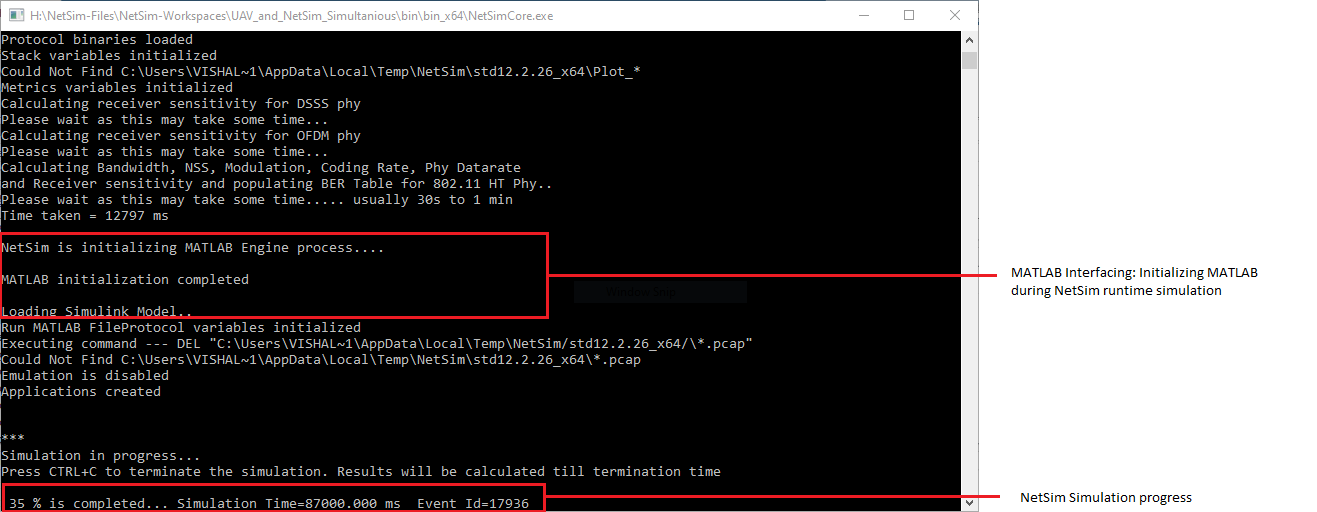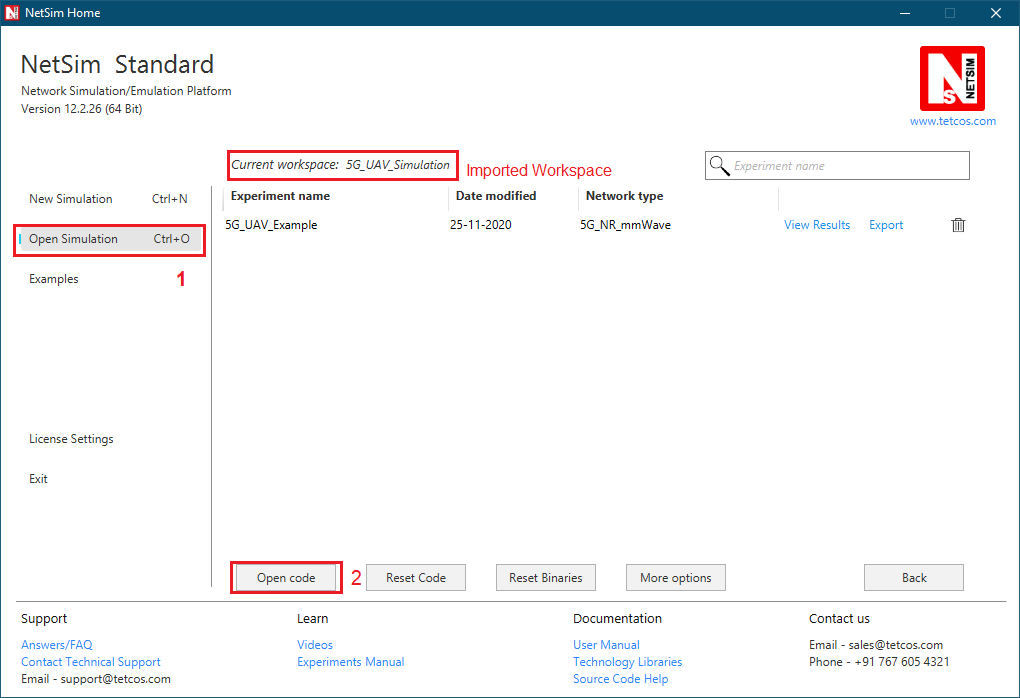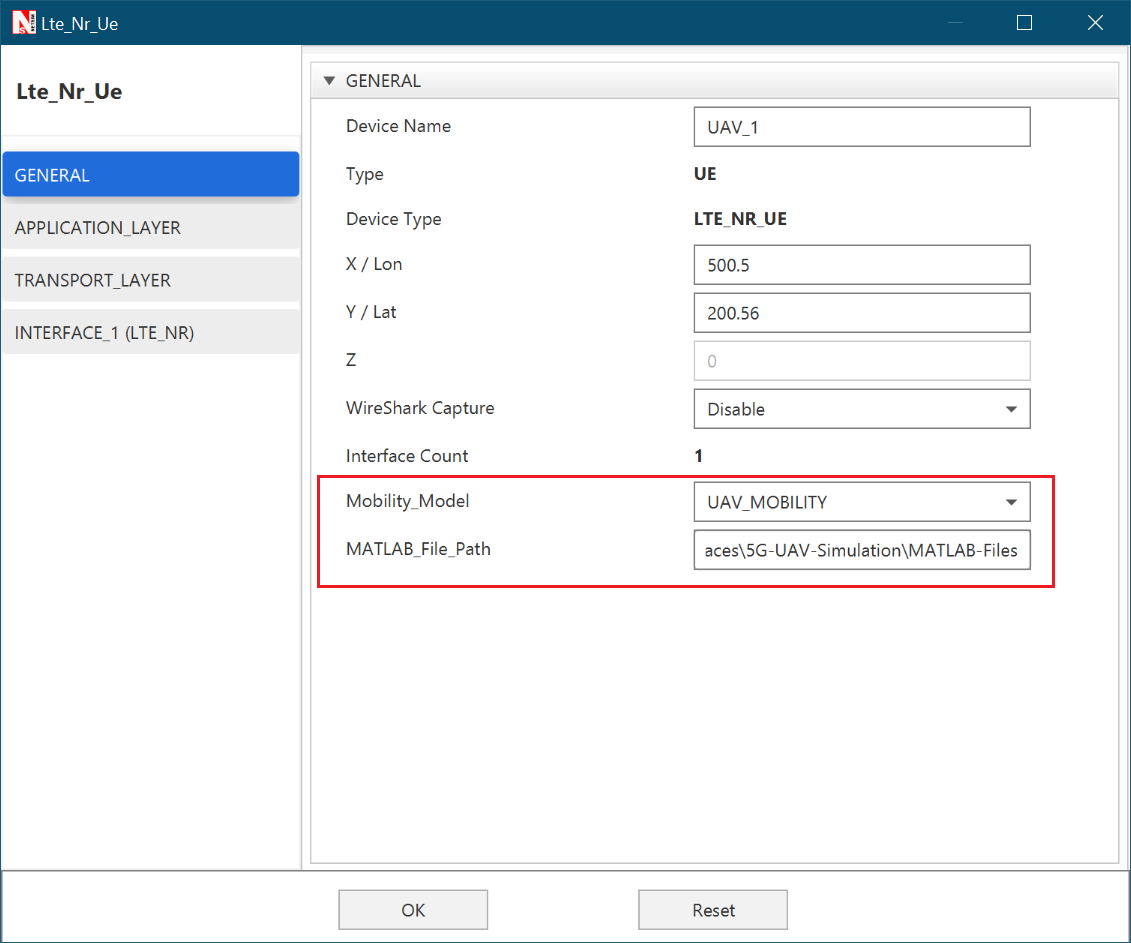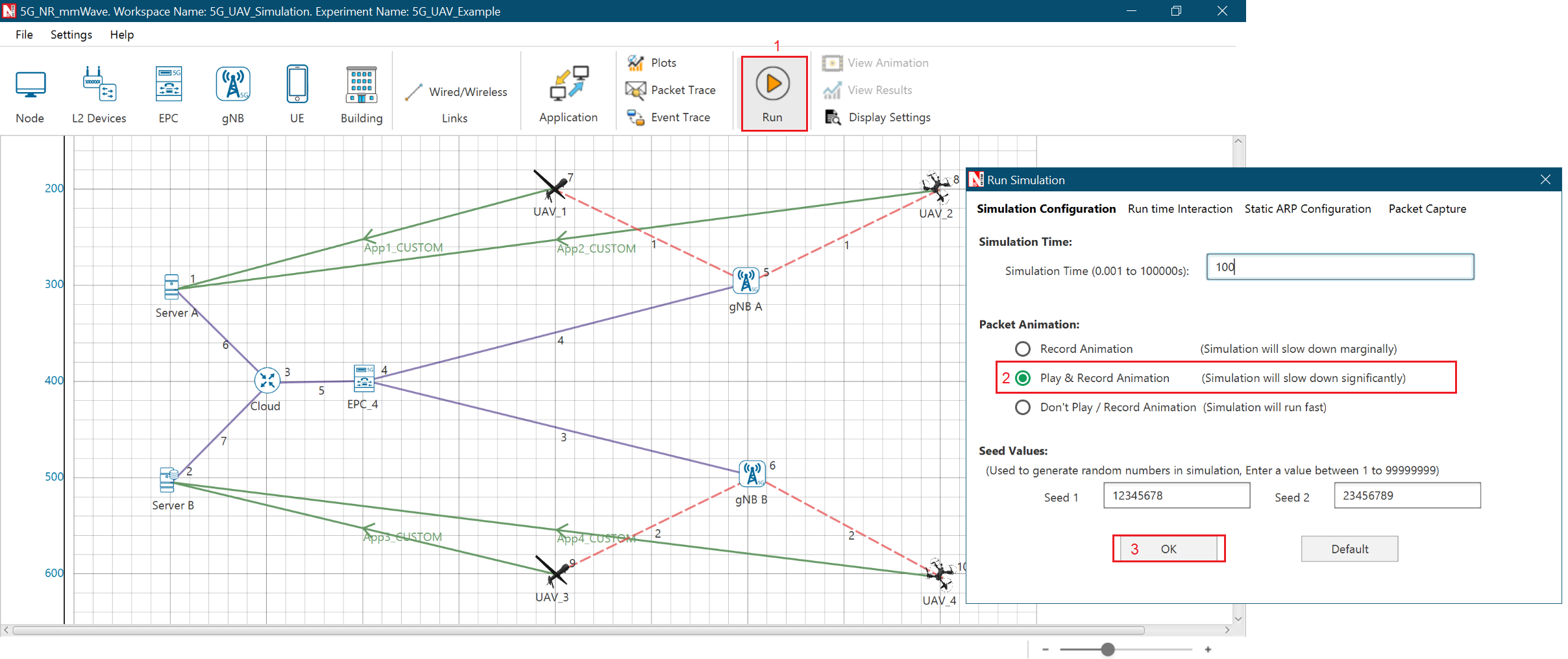Applicable Versions NetSim Standard NetSim Pro

 Applicable Releases v12.2 v13.2

 MATLAB Version R2019b or above MATLAB Toolbox MATLAB, Simulink, Robotics and System Toolbox File Exchange Project of MATLAB Robotics System Toolbox UAV Library Visual Studio Community Edition

#### Objective

To simulate the UAVs/drones in NetSim by interfacing with MATLAB's Simulink.

#### 3D View in MATLAB and 2D View in NetSim2D- Animation in NetSim 3D Animation in MATLABNetSim Simulation Window

https://tetcos.freshdesk.com/support/solutions/articles/14000099351-how-to-clone-netsim-fileexchange-project-repositories-from-github

Other tools such as GitHub Desktop, SVN Client, Sourcetree, Git from the command line, or any client you like to clone the Git repository.

 5G UAV Project - NetSim v13.2 5G UAV Project - NetSim v12.2(NetSim Workspace)

`Note: It is recommended not to download the project as an archive (compressed zip) to avoid incompatibility while importing workspaces into NetSim.`

Upon interfacing NetSim with MATLAB the following tasks are performed during simulation start:

• MATLAB Engine process is initialized
• MATLAB Desktop window is loaded

Upon simulating a network created in NetSim the following tasks are performed periodically:

• Appends the readings to the packet payload as packets are formed

During the Simulation, the SIMULINK Model is started and paused several times for NetSim and SIMULINK simulations to run synchronously. The X, Y and Z coordinates obtained from SIMULINK are read from MATLAB workspace and given as input to NetSim's Mobility model. In this example, coordinates are taken every one second and updated to the device mobility.

#### Output/Metrics specific to this example

• NetSIm Animation- Mobility of the devices configured in NetSim is given as input from MATLAB

#### Modifications done to NetSim Source codes:

Project: Mobility

Files

• Mobility.c,
• Mobility.h,
• Mobility.vcxproj (Project file)

#### Sections of source code modified

• Mobility.c
• fn_NetSim_Mobility_Init(): call to init_uav() function
• fn_NetSim_Mobility_Run(): Call to uav_run() function
• fn_NetSim_Mobility_Finish()
• Mobility.h
• MATLAB Engine variable - Used to initiate and interact with MATLAB Engine process
• Mobility.vcxproj - This is a Visual Studio project file used to load and manage the source codes related to the Mobility in NetSim
• path to MATLAB application
• path to MATLAB include directory
• path to MATLAB lib directory
• information related to dependent MATLAB library files
• UAVBasedMobility.c
• init_uav():  Initializes MATLAB, Loads SIMULINK Model, starts and pauses SIMULINK simulation, and initializes the UAV devices in MATLAB to start simulation along with NetSim's simulation.
• uav_run(): Starts NetSim and MATLAB simulation simultaneously and gets the co-ordinates from MATLAB workspace for every step size set in NetSim.
• uavcorr():  Function to get co-ordinates from MATLAB.

#### Procedure to setup MATLAB for this example

• Create a system environment variable with name as "MATLAB_PATH" and value as "<path of MATLAB Root directory>"Note: The procedure to create an environment variable and the path of the MATLAB root directory will vary based on the Windows OS version and the MATLAB software version respectively.

#### Procedure to setup NetSim for this example

• Clone the project using the Git-Hub link provided with the beginning of this article. And here is the procedure on How to clone NetSim File Exchange project repositories from GitHub.
• Import the workspace to NetSim. Refer Chapter 4, Section 4.9 of User Manual.
• Place MATLAB files in a system location with both read and write permissions.
• Backup and Replace GenerlProperty.xml file provided in NetSim-Files folder in the cloned project to <NetSim-Installation-Directory>/Docs/XML/Properties folder.
• Open code from NetSim Home Screen. (NetSim Home Screen > Open Simulation > Open code)• Rebuild the code#### Running the NetSim scenario with UAV Based Mobility

• Before opening,, makes sure that you have replaced the XML file in the NetSim Installation directory mentioned above and then Open Example saved in the workspace.
• Make sure that all UAV/Drone/UE's are UAV Mobility enabled and the path to MATLAB files that you downloaded with the attachment
• Also, make sure that you provide the path using single inverted commas. ('<Matlab Files Path>')• Run the simulation with Play and record animation in order to have both Animations simultaneously• MATLAB Animation• End of Simulation:#### Code modifications in NetSim

A new file UAVBasedMobility.c is added to the Mobility Project, which contains the following source code:

`#include "main.h"#include "Mobility.h"#include "Animation.h"double* uavcorr(char* id);char* matlabloc;/*init_uav() function is to initialize MATLAB Engine and start UAVs*/void init_uav(){	static int nouav = 1;	// Declared as static, since we want it to be declared and changed only once		char buf = "cd ";	char wp;	char sim = "opSimulink";	strcpy(wp, matlabloc);	strcat(buf, wp);		if (nouav)					// This will run only at the 1st time	{		//MATLAB/SIMULINK INTERFACING		fprintf(stderr, "\nNetSim is initializing MATLAB Engine process....\n");		if (!(ep = engOpen(NULL))) {			MessageBox((HWND)NULL, (LPCWSTR)"Can't start MATLAB engine",(LPCWSTR)"MATLAB_Interface.c", MB_OK);			fprintf(stderr, "\nMATLAB Initialization Failed\nPress any key to proceed without MATLAB...\n");			_getch();		}		else		{			engEvalString(ep, "desktop");			engEvalString(ep, buf); //Update user-path			fprintf(stderr, "\nMATLAB initialization completed\n");			fprintf(stderr, "\nLoading Simulink Model..");			engEvalString(ep, sim);		}		//MATLAB/SIMULINK INTERFACING		nouav = 0;	}}/*This function is used to receive co-ordinates from MATLAB during run time*/void uav_run(){	MOBILITY_VAR* pstruMobilityVar = (MOBILITY_VAR*)NETWORK->ppstruDeviceList[pstruEventDetails->nDeviceId - 1]->pstruDeviceMobility->pstruMobVar;	//Define Mobility variable	double dPresentTime = pstruMobilityVar->dLastTime;	memcpy(NETWORK->ppstruDeviceList[pstruEventDetails->nDeviceId - 1]->pstruDeviceMobility->pstruCurrentPosition,		NETWORK->ppstruDeviceList[pstruEventDetails->nDeviceId - 1]->pstruDeviceMobility->pstruNextPosition,		sizeof * NETWORK->ppstruDeviceList[pstruEventDetails->nDeviceId - 1]->pstruDeviceMobility->pstruCurrentPosition);	if (pstruMobilityVar->dLastTime + pstruMobilityVar->dPauseTime * 1000000 < pstruEventDetails->dEventTime + 1000000)	//Everytime Mobility being called	{		double* coordinates;		// Pointer for array of X and Y coordinates		coordinates = uavcorr(pstruEventDetails->nDeviceId);	//Get coordinates from matlab		if (coordinates != NULL)		{			NETWORK->ppstruDeviceList[pstruEventDetails->nDeviceId - 1]->pstruDeviceMobility->pstruNextPosition->X = coordinates;	// Update the coordinates in Network stack			NETWORK->ppstruDeviceList[pstruEventDetails->nDeviceId - 1]->pstruDeviceMobility->pstruNextPosition->Y = coordinates;			NETWORK->ppstruDeviceList[pstruEventDetails->nDeviceId - 1]->pstruDeviceMobility->pstruNextPosition->Z = coordinates;			free(coordinates);			// Free memory of pointer		}		//store the last time		pstruMobilityVar->dLastTime = pstruEventDetails->dEventTime + 100;			// Update Last time since we want to match timings with MATLAB	}	//update the device position	memcpy(NETWORK->ppstruDeviceList[pstruEventDetails->nDeviceId - 1]->pstruDevicePosition,		NETWORK->ppstruDeviceList[pstruEventDetails->nDeviceId - 1]->pstruDeviceMobility->pstruCurrentPosition,		sizeof * NETWORK->ppstruDeviceList[pstruEventDetails->nDeviceId - 1]->pstruDevicePosition);	mobility_pass_position_to_animation(pstruEventDetails->nDeviceId,		pstruEventDetails->dEventTime,		DEVICE_POSITION(pstruEventDetails->nDeviceId));	//Add event for next point 	pstruEventDetails->dEventTime += (1* SECOND);	fnpAddEvent(pstruEventDetails);	pstruEventDetails->dEventTime -= (1 * SECOND);}	double* uavcorr(int id){	double* coordinates;	char buf;	mxArray* xmat = NULL;	mxArray* ymat = NULL;	mxArray* zmat = NULL;	double* xcor = NULL;	double* ycor = NULL;	double* zcor = NULL;	if (id == 1)		engEvalString(ep, "set_param('UAV1','SimulationCommand','continue');set_param('UAV1','SimulationCommand','pause');");	else if (id == 2)		engEvalString(ep, "set_param('UAV2','SimulationCommand','continue');set_param('UAV2','SimulationCommand','pause');");	else if (id == 3)		engEvalString(ep, "set_param('UAV3','SimulationCommand','continue');set_param('UAV3','SimulationCommand','pause');");	else		engEvalString(ep, "set_param('UAV4','SimulationCommand','continue');set_param('UAV4','SimulationCommand','pause');");				engEvalString(ep, "[xa,c]=size(North)");	engEvalString(ep, "x_out = North(xa, :)");	engEvalString(ep, "[ya, c] = size(East)");	engEvalString(ep, "y_out = East(ya, :)");	engEvalString(ep, "[za, c] = size(Height)");	engEvalString(ep, "z_out = Height(za, :)");	xmat = engGetVariable(ep, "x_out");	ymat = engGetVariable(ep, "y_out");	zmat = engGetVariable(ep, "z_out");	xcor = mxGetPr(xmat);	ycor = mxGetPr(ymat);	zcor = mxGetPr(zmat);	coordinates = (double*)malloc(3 * sizeof * coordinates);	coordinates = xcor;	coordinates = ycor;	coordinates = zcor;	return (coordinates);}`

`% This function is used to initialize and start Simulink model called in NetSim- init_uav() function.% Initiating UAV 1model='UAV1';load_system(model);set_param(model,'SimulationCommand','start')set_param(model,'SimulationCommand','pause')% Initiating UAV 2model1='UAV2';load_system(model1);set_param(model1,'SimulationCommand','start')set_param(model1,'SimulationCommand','pause')% Initiating UAV 3model2='UAV3';load_system(model2);set_param(model2,'SimulationCommand','start')set_param(model2,'SimulationCommand','pause')% Initiating UAV 4model3='UAV4';load_system(model3);set_param(model3,'SimulationCommand','start')set_param(model3,'SimulationCommand','pause')`